## Saturday, April 1, 2017

### On Thermal Expansion & Thermal Contraction - 16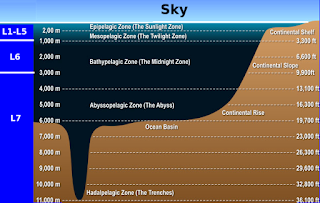Fig. 1 Ocean Depth Levels
"Why do you rob banks?", the notorious bank robber was asked, "Because that is where the money is," she replied.

"Why does Dredd Blog have a '>3000m' level in its graphs and reports" the notorious blogger was asked, "Because that is where the water is" answered the blogger.

"The average depth of the ocean is about 12,100 feet [3,688 m] " (NOAA), and some 90% of ocean water is below 200m.

The modified graphic at Fig. 1 (see link there) shows the depth levels used by Dredd Blog.

They are designated as "L1-L7", which equate to:
L1    0-200 m
L2    201-400 m
L3    401-600 m
L4    601-800 m
L5    801-1000 m
L6    1001-3000 m
L7    >3000 m
In other words, Dredd Blog analysis uses World Ocean Database measurements from every depth measured and placed in the CTD and PFL datasets (Databases Galore - 18).

Some scientists issue a caution about anything less:
"Despite recent advances in the state of the global ocean observing system, estimating oceanic variability on basin-wide to global scales remains difficult. Errors in such estimates can be large and often go unreported in the literature. In order to make the most accurate estimates of oceanic variability, it is necessary to combine different types of data into a single consistent field."
(Willis et al. 2004, J. Geophys. Res., 109, PDF). One of the IPCC editors/authors, Nathan Bindoff, indicates:
"The data used for temperature and heat content estimates are based on the World Ocean Database 2001." - p. 389
...
"Approximately 7.9 million temperature profiles were used in constructing the two longer time series."- p. 390
(Bindoff et al., 2007, PDF). That is a robust number of measurements but it leaves out the deeper waters:
"Figure 5.1. Time series of global annual ocean heat content (1022 J) for the 0 to 700 m layer." - p. 390
...
"Figure 5.2. Linear trends (1955–2003) of change in ocean heat content per unit surface area (W m–2) for the 0 to 700 m layer..." - p. 391
(ibid, Bindoff et al. 2007). Regular readers of Dredd Blog know that I use measurements from the World Ocean Database to analyze temperature and salinity measurements at all depths for which measurements have been taken and recorded.

Those data are arranged in various configurations (year, day-of-year, and month) then combined, as suggested by the scientist quoted above who wrote:
"In order to make the most accurate estimates of oceanic variability, it is necessary to combine different types of data into a single consistent field."
(ibid, Willis et al. 2004). That is what I like to do too (On Thermal Expansion & Thermal Contraction - 15).

If one wants to analyze all of the ocean, then all of the measurements available should be used.

This is not always the case, and in fact the practice seems to be to only use measurements in the upper half, or less, of the total ocean depths.

Here is one reason why it is important to consider the generally colder, deeper water:
"Sea level will rise if the ocean warms and fall if it cools, since the density of the water column will change." - p. 414
...
"Global ocean heat content observations show considerable interannual and inter-decadal variability superimposed on the longer-term trend. Relative to 1961 to 2003, the period 1993 to 2003 has high rates of warming but since 2003 there has been some cooling." - p. 387
(ibid, Bindoff et al. 2007, emphasis added). In other words, as I have written over and over, there is both "thermal contraction" and "thermal expansion" (On Thermal Expansion & Thermal Contraction)..

Yet, sea level is not rising or falling because thermal expansion and contraction are the major factors (The Bathtub Model Doesn't Hold Water, 2, 3, 4).

Hopefully, the IPCC establishment scientists will discover the ghost water they have been utterly clueless about, because like its source gravity, it is especially invisible when we are not looking for it (The Ghost-Water Constant, 2, 3, 4, 5, 6, 7; The Gravity of Sea Level Change, 2, 3, 4).

If they stop scratching the surface and really look close into the depths, they will find out why the surface can cool some without a decrease in overall heat quantity contained in the oceans:
"The oceans are warming. Over the period 1961 to 2003, global ocean temperature has risen by 0.10°C from the surface to a depth of 700 m. Consistent with the Third Assessment Report (TAR), global ocean heat content (0–3,000 m) has increased during the same period, equivalent to absorbing energy at a rate of 0.21 ± 0.04 W m–2 globally averaged over the Earth’s surface. Two-thirds of this energy is absorbed between the surface and a depth of 700 m. Global ocean heat content observations show considerable interannual and inter-decadal variability superimposed on the longer-term trend. Relative to 1961 to 2003, the period 1993 to 2003 has high rates of warming but since 2003 there has been some cooling."
(ibid, Bindoff et al. 2007, emphasis added). Those upper levels can 'cool' even as the overall ocean heat content increases.

Why? ("The first statement of the 2nd law of thermodynamics - heat flows spontaneously from a hot to a cold body ..." - On Thermal Expansion & Thermal Contraction - 9).

Some of the heat flows from the shallower depth levels they tend to solely focus on (<700 m) into the deeper waters (>3000m) which is where the most ocean water is.

The amount of heat in the ocean is not diminished just because some of that heat moves out of those shallower depths and into the deeper depths.

But, the temperature will drop at depths where the heat flows away from those shallower depths (<700 m) into deeper, cooler water (>3000m, where the temperature will rise unnoticed unless it is measured).

Remember that the changes I reported in the previous post of this series are about changes in temperature and salinity taking place at various depths at various latitude layers.

It is not about a decrease of heat content in the oceans.

The reality is that temperatures and salinity at one depth will change as the heat leaves that depth and flows to a deeper depth, without any diminution of the total ocean heat content.

The next post in this series is here, the previous post in this series is here.

## Thursday, March 30, 2017

### On Thermal Expansion & Thermal Contraction - 15

Regular readers know (as this series shows) that I am "on the case" in the matter of the hypothesis which asserts that "thermal expansion is the major cause of sea level rise in the 20th and 21st centuries".

I can tell you that I have now completed the modules that process all layers of the World Ocean Database (WOD), a database containing billions of measurements taken by hundreds of scientists over many years (see e.g. Databases Galore - 18).

The newly completed modules are NOT for creating graphs.

They are for using the (~0.97 billion measurements) I have used to make SQL databases for detecting changes in temperature and salinity in every depth level of every square mile of every ocean on planet Earth (where WOD measurements have been taken and recorded).

These final product of these three modules is a detailing of changes, and the net result of such changes in terms of increase or decrease in temperature and in salinity of ocean water.

The process focuses on "latitude layers" which are simply eighteen bands of ten degrees latitude in breadth, spanning the entire globe.

They meet at the Equator (see The Layered Approach To Big Water - 8 for a look at the Northern Hemisphere layers).

II. The Process

First I gather the measurements by year, by month, and by day of year.

The year-data is the only one that has a variable number of final components based upon which years in which data was gathered.

The month-data has a fixed number of final components, 12, one for each month.

The day-of-year-data also has a fixed number of final components, 366, one for each day of the year, including leap years.

The software modules load rows of data from an SQL server to generate a mean average of all measurements for each module (i.e. by-year module, by-day-of-year module, and by-month module).

As you might surmise, the by-year module focuses on detecting temperature changes (warming & cooling) year by year; the by-day-of-year modules focuses on detecting temperature changes (warming & cooling) day to day; and the by-month module focuses on detecting temperature changes (warming & cooling) month by month.

They ignore measurements that are not an increase or decrease in temperature and salinity compared to the previous measurement they analyzed.

In other words, depending on the module, if two sequential years, days, or months have the same mean average, there is no change, so depending on the module, the process moves to the next year, day, or month.

Changes are recorded as increases and decreases as the process traverses the data, until the last measurement is processed, then a compilation is generated.

I will have enough to bore you before all is said and done, because there are eighteen layers times 366 + eighteen layers times 12, and eighteen layers times x years to consider.

Fortunately, I  did make a section in each report where the full layer values are recorded, which is much smaller in terms of data, than the entire report.

III. An Example

Following is an example printout of LAYER EIGHT from the by-month module (NOTE: L1 = 0-200m, L2 = 201-400m, L3 = 401-600m, L4 = 601-800m, L5 = 801-1000m, L6 = 1001-3000m, and L7 = >3000m):

Month Report (Temperature & Salinity)

month = 1

Temperature Values (mean avg.):
L1= 14.0434, L2= 10.4838, L3= 7.97412, L4= 6.43355, L5= 5.51067, L6= 3.46801, L7= 1.0671

Salinity Values (mean avg.):
L1= 29.9163, L2= 33.1275, L3= 32.6764, L4= 31.9657, L5= 31.8679, L6= 31.9872, L7= 14.0654

month = 2

Temperature Values (mean avg.):
L1= 14.8087, L2= 10.6887, L3= 8.15225, L4= 6.44354, L5= 5.33347, L6= 3.64096, L7= 1.09391

Salinity Values (mean avg.):
L1= 30.9779, L2= 34.2648, L3= 34.2777, L4= 33.9782, L5= 33.916, L6= 33.8689, L7= 16.8543

month = 3

Temperature Values (mean avg.):
L1= 14.0587, L2= 10.613, L3= 7.91122, L4= 6.52757, L5= 5.43234, L6= 3.83356, L7= 1.10093

Salinity Values (mean avg.):
L1= 30.7209, L2= 34.0975, L3= 34.2926, L4= 34.1917, L5= 34.2082, L6= 33.4592, L7= 15.7349

month = 4

Temperature Values (mean avg.):
L1= 15.273, L2= 11.0116, L3= 8.246, L4= 6.63033, L5= 5.58023, L6= 3.78207, L7= 1.10204

Salinity Values (mean avg.):
L1= 31.2721, L2= 34.4654, L3= 33.9041, L4= 33.7741, L5= 34.0265, L6= 33.2908, L7= 16.4524

month = 5

Temperature Values (mean avg.):
L1= 14.5128, L2= 10.7849, L3= 8.09729, L4= 6.52235, L5= 5.64661, L6= 3.85766, L7= 0.995954

Salinity Values (mean avg.):
L1= 30.0223, L2= 33.8994, L3= 33.5211, L4= 33.2569, L5= 33.2614, L6= 33.0917, L7= 12.1004

month = 6

Temperature Values (mean avg.):
L1= 13.0053, L2= 10.4697, L3= 7.77111, L4= 6.28189, L5= 5.29967, L6= 3.53573, L7= 1.05035

Salinity Values (mean avg.):
L1= 30.198, L2= 33.8953, L3= 33.0271, L4= 33.4587, L5= 33.2884, L6= 33.7094, L7= 15.3025

month = 7

Temperature Values (mean avg.):
L1= 13.8094, L2= 10.7479, L3= 8.22236, L4= 6.47902, L5= 5.5412, L6= 3.96159, L7= 1.23292

Salinity Values (mean avg.):
L1= 30.7863, L2= 33.2537, L3= 33.2177, L4= 33.1016, L5= 33.3766, L6= 33.4571, L7= 17.6366

month = 8

Temperature Values (mean avg.):
L1= 14.1374, L2= 10.6184, L3= 7.97364, L4= 6.3522, L5= 5.36583, L6= 3.63349, L7= 1.34836

Salinity Values (mean avg.):
L1= 32.1701, L2= 33.9175, L3= 33.6309, L4= 33.5921, L5= 33.5165, L6= 33.7212, L7= 19.7673

month = 9

Temperature Values (mean avg.):
L1= 12.9352, L2= 10.4553, L3= 7.8871, L4= 6.55954, L5= 5.76661, L6= 3.72202, L7= 1.15517

Salinity Values (mean avg.):
L1= 30.5745, L2= 33.3848, L3= 33.3058, L4= 33.5662, L5= 33.9286, L6= 33.016, L7= 14.4325

month = 10

Temperature Values (mean avg.):
L1= 13.3937, L2= 10.8998, L3= 8.08434, L4= 6.41589, L5= 5.40362, L6= 3.71293, L7= 1.1296

Salinity Values (mean avg.):
L1= 29.5143, L2= 33.5229, L3= 33.2096, L4= 32.9099, L5= 33.4544, L6= 32.8567, L7= 14.4878

month = 11

Temperature Values (mean avg.):
L1= 14.2476, L2= 10.4884, L3= 7.71465, L4= 6.22846, L5= 5.15675, L6= 3.53236, L7= 1.07716

Salinity Values (mean avg.):
L1= 28.9627, L2= 33.3538, L3= 32.0359, L4= 33.5587, L5= 32.7503, L6= 33.0735, L7= 12.9157

month = 12

Temperature Values (mean avg.):
L1= 13.6677, L2= 10.4135, L3= 8.01104, L4= 6.5214, L5= 5.34149, L6= 3.53923, L7= 1.05744

Salinity Values (mean avg.):
L1= 30.1674, L2= 34.5667, L3= 33.8299, L4= 33.3359, L5= 33.5153, L6= 34.1303, L7= 16.0656

By Month Layer Totals (Temperature & Salinity)

Temperature Changes:

Increases:
L1= 0.737358, L2= 0.331592, L3= 0.291562, L4= 0.14903, L5= 0.190034, L6= 0.160397, L7= 0.064557

Decreases:
L1= 0.95996, L2= 0.199524, L3= 0.236815, L4= 0.161266, L5= 0.261878, L6= 0.178233, L7= 0.0794003

Net Layer Temperature Change (all depths): -0.152546

Salinity Changes:

Increases:
L1= 0.827567, L2= 0.70396, L3= 0.8028, L4= 0.7134, L5= 0.538936, L6= 0.80743, L7= 2.05408

Decreases:
L1= 0.94284, L2= 0.346775, L3= 0.476758, L4= 0.36615, L5= 0.531263, L6= 0.315675, L7= 3.09456

Net Layer Salinity Change (all depths): 0.374149

IV. Another Example

The software prints a report, a compilation of all layers that the software module processed as follows (this is also a report of the by-month module) UPDATE: this is all layers, all types, in HTML:

Summary Report - All Layers
(by month, year, day of year)

Change @ Layer 0

By day-of-year:
Temperature: -0.249233
Salinity: 0.0357515

By Month:
Temperature: 0.148802
Salinity: -1.93054

By Year:
Temperature: 0.730141
Salinity: -9.30913

Change @ Layer 1

By day-of-year:
Temperature: -0.20873
Salinity: 0.352097

By Month:
Temperature: -0.288768
Salinity: -1.05714

By Year:
Temperature: -1.02197
Salinity: 6.39866

Change @ Layer 2

By day-of-year:
Temperature: 0.226395
Salinity: 0.321025

By Month:
Temperature: 0.668749
Salinity: -2.1037

By Year:
Temperature: 0.0147982
Salinity: 2.07279

Change @ Layer 3

By day-of-year:
Temperature: 0.0701951
Salinity: -0.0988995

By Month:
Temperature: 0.161305
Salinity: -0.351641

By Year:
Temperature: 0.292753
Salinity: -1.16898

Change @ Layer 4

By day-of-year:
Temperature: -0.0639118
Salinity: -0.272323

By Month:
Temperature: 0.304104
Salinity: 0.250932

By Year:
Temperature: 0.974211
Salinity: 1.24173

Change @ Layer 5

By day-of-year:
Temperature: 0.0231685
Salinity: 0.234071

By Month:
Temperature: -0.112422
Salinity: 0.516272

By Year:
Temperature: -0.227686
Salinity: -0.159992

Change @ Layer 6

By day-of-year:
Temperature: -0.0387131
Salinity: -0.19058

By Month:
Temperature: -0.340361
Salinity: -0.136017

By Year:
Temperature: -1.66551
Salinity: -1.95515

Change @ Layer 7

By day-of-year:
Temperature: -0.00697605
Salinity: -0.00730471

By Month:
Temperature: -0.330484
Salinity: -1.50395

By Year:
Temperature: -0.828885
Salinity: -2.06076

Change @ Layer 8

By day-of-year:
Temperature: -0.0388297
Salinity: 0.124951

By Month:
Temperature: -0.152546
Salinity: 0.374149

By Year:
Temperature: 0.968501
Salinity: 2.0629

Change @ Layer 9

By day-of-year:
Temperature: -0.0146486
Salinity: -0.0120359

By Month:
Temperature: -0.124228
Salinity: 0.656732

By Year:
Temperature: -0.0776117
Salinity: -3.34536

Change @ Layer 10

By day-of-year:
Temperature: -0.0446372
Salinity: -0.518755

By Month:
Temperature: 0.329829
Salinity: 0.311527

By Year:
Temperature: 1.10711
Salinity: 1.45228

Change @ Layer 11

By day-of-year:
Temperature: 0.0436973
Salinity: -0.278299

By Month:
Temperature: -0.0952014
Salinity: -0.267137

By Year:
Temperature: -0.692185
Salinity: -1.3157

Change @ Layer 12

By day-of-year:
Temperature: 0.0239367
Salinity: 0.174405

By Month:
Temperature: -0.0159302
Salinity: -0.292348

By Year:
Temperature: -1.50679
Salinity: -0.784702

Change @ Layer 13

By day-of-year:
Temperature: 0.0368379
Salinity: 0.0226267

By Month:
Temperature: -0.103631
Salinity: -1.05413

By Year:
Temperature: 0.855217
Salinity: -2.88072

Change @ Layer 14

By day-of-year:
Temperature: 0.021951
Salinity: 0.132577

By Month:
Temperature: 0.0391302
Salinity: -0.0457825

By Year:
Temperature: 0.785626
Salinity: 0.421682

Change @ Layer 15

By day-of-year:
Temperature: -0.0331067
Salinity: -0.413596

By Month:
Temperature: -0.249408
Salinity: -1.68247

By Year:
Temperature: -0.262004
Salinity: 2.63629

Change @ Layer 16

By day-of-year:
Temperature: -0.448942
Salinity: -0.535457

By Month:
Temperature: 0.299387
Salinity: -0.288502

By Year:
Temperature: 0.627145
Salinity: -0.452906

Change @ Layer 17

By day-of-year:
Temperature: 0
Salinity: 0

By Month:
Temperature: 0
Salinity: 0

By Year:
Temperature: 0
Salinity: 0

Change Sub-Totals

By-DOY Temperature Change: -0.701547
By-DOY Salinity Change: -0.929748

By-Month Temperature Change: 0.138326
By-Month Salinity Change: -8.60373

By-Year Temperature Change: 0.0728571
By-Year Salinity Change: -7.14707

Change Totals

Temperature Change: -0.163455
Salinity Change: -5.56018

As you can see, the thermal expansion hypothesis is in trouble.

Considering those ~0.97 billion WOD records (about 5% was rejected by the modules), using the CTD and PFL datasets, we find that all layers at all depths in the by-month view in all three views show that the ocean water has warmed changed a mean-average of 0.138326 degrees C -0.163455 degrees C since those measurements began to be recorded.

The degree of salinity dropped changed a mean-average of ~8.6 -5.56018.

All of which does not add up to "thermal expansion is the major cause of sea level rise in the 20th and 21st centuries"  (Humble Oil-Qaeda).

VI. More Work To Be Done

I have a suspicion that the thermal expansion "rumor" was started by scientists who are a bit too close to the oil industry.

As of yet I have no documentation sequestered from the vast collection of documents leading to my suspicion (Smoke & Fumes).

VII. Conclusion

There is a lot of work still to be done.

If you know of any leads, share your comments so as to help others who are with us.

The next post in this series is here, the previous post in this series is here.

## Sunday, March 26, 2017

### The Layered Approach To Big Water - 8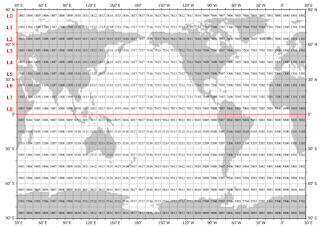Fig. 1 N. Hemisphere Layers Zero - Layer Nine
I. A College Experiment

Today, along with the graphs of N. Hemisphere Layer Zero through Layer Eight (in Month format), let's peruse a college level class guidance sheet concerning the hypothesis of thermal expansion being the major cause of sea level rise in the 19th and 20th centuries (Oregon State, PDF).

This material is taught to college / university level students who put on their lab coats and do the following experiment.

First, a 125-250 ml. conical flask is filled with water.

The flask is then equipped with a two hole cork, which is placed into the opening at the top, and then two thin glass tubes are forced into the holes in the cork.Fig. 2 Layer Eight
This is supposed to represent the deep and wide oceans (it doesn't).

II. A Better Experiment

For this experiment scenario to be an accurate representation of ocean heating, it would have to be a very deep flask, say 20-30 ft., filled with ocean water of different temperatures and salinity levels (and a certain percentage of its lower area should be coated or wrapped with black paper, tape, or plastic to represent the cold, dark depths of the ocean.Fig. 3 Layer Seven
The lower level (representing >3000 m) should be at a temperature from about 5 deg. C down to less than 0 deg. C, depending on which layer (Fig. 1, L0-L8) is being simulated.

The upper layers should typically have graduated temperatures, generally warmer as the surface is approached (see Fig. 2 - Fig. 4).

The heat applied via a lamp (simulating sunlight striking the surface) should impact the surface area only.Fig. 4 Layer Six

Absent this more realistic lab setup, this experiment at Oregon State, typical of college labs, shows how a myth is advanced.

Anyway, when the warmth in the light impacting the water at the surface of the flask increases (simulating sunlight entering the ocean at the surface), the second law of thermodynamics will apply, and the heat in the warmer water will flow to the cooler water, as equilibrium is sought to be attained.

This applies in a manner that causes heat flow horizontally and/or vertically, depending on the current conditions in that area.

Even with the better experiment, the class will wait all semester, or longer, before any meaningful net thermal expansion takes place.Fig. 5 Layer Five
III. The Best Experiment

The best "experiment" is to OBSERVE the actual measurements, taken by research scientists over the years, then placed into the World Ocean Database (WOD).

There are billions of such measurements (I myself have personally downloaded ~0.97 billion such measurements to use for generating graphs (Databases Galore - 18).

Such observations shown in graphs in this post, and in other Dredd Blog series, reveal that even though most of the heat (~95%) being trapped by greenhouse gases is making its way into the oceans, it does not result in a dynamic that is "the major or a major cause of sea level rise due to thermal expansion."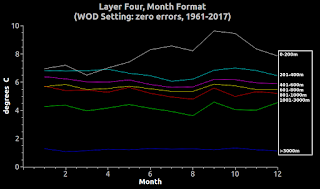Fig. 6 Layer Four
What does take place is that the distance between the lines on the graph, which represent temperature levels, will increase and decrease as the laws of thermodynamics and fluid dynamics apply.

The warmth or heat entering the water at the surface flows toward and into colder water (over, under, and/or beside that warming water).

This thermodynamic flow, in general, will be in a downward direction (deeper water) until a "contextual equilibrium" is reached.Fig. 7 Layer Three
At that time the status quo will emerge and persist until more heat is added by more sunlight (at which time the cycle repeats, forcing the temperature increase downward until the warmer water drops 'x' amount as the lower level temperature increases 'x' amount).

I wrote "contextual equilibrium" because various factors such as salinity, currents, and several other factors can resist or halt the flow for a time.

Nevertheless, when the ocean wakes up in the morning to start all over again, the laws of fluids and thermodynamics will still be around.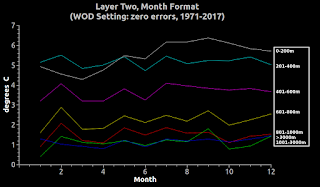Fig. 8 Layer Two
IV. A Discussion of the Class Discussion

The teacher is guided by the Oregon State guidance sheet.

The text reads "If global temperature increases, many scientists have indicated that an increase in sea level is one of the most likely secondary effects." (p. 1)

It may be true that "many scientists have indicated," but many of that "many" do so parroting previous statements rather than "going to the lab" themselves.

Actually, the "most likely secondary effects" are dying coral, extinction of species, and increasing temperature measurements further down in the ocean depths.Fig. 9 Layer One
Once contextual equilibrium is reached in an ocean area, then thermal expansion becomes a candidate for a minor cause, not a major cause, of sea level rise.

But as soon as the context changes in that area, such as salinity levels, then the thermodynamic transfer of warm to cold kicks in once again and the temperature at the warmer level drops as the temperature at the cooler level increases until a contextual equilibrium is reached (On Thermal Expansion & Thermal Contraction, 2, 3, 4, 5, 6, 7, 8, 9, 10, 11, 12, 13, 14).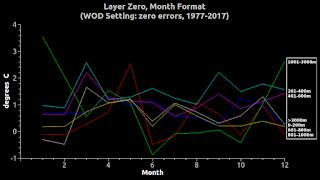Fig. 10 Layer Zero
But more than that, if that contextual temperature had been sufficient to cause sea level rise there in that contextual area, the sea level will fall the amount it had risen (thermal contraction) when the temperature there drops (ibid).

Nevertheless, when the status quo temperature at any contextual area is harmful to species in that water, damage is going to be done regardless of the advent, or not, of thermal expansion induced sea level rise or thermal contraction induced sea level fall.

V. The Big Picture

The teacher's guide goes on to indicate: "Second, rising temperatures will cause the ice and snowfields to melt, thereby increasing the amount of water in the oceans" (first came ice-melt induced sea level fall around Greenland, and redistribution of that melt-water toward the equator - Proof of Concept - 3).

What would the teacher say if a student were to ask "how much sea level can thermal expansion cause compared to ice melting?"

Would the teacher refer to NOAA et al. calculations that the maximum amount of sea level rise caused by ice melt is about 80.32 meters or 263.51 feet (USGS) ?

Or would she refer to the Dredd Blog calculation based on ghost water (The Ghost-Water Constant, 2, 3, 4, 5, 6, 7) ?

Or would he say it depends on where you would measure the change, because, due to gravitational and rotational dynamics it is not like what would take place in a bathtub (The Gravity of Sea Level Change, 2, 3, 4) ?

VI. Conclusion

We have to trust (The Pillars of Knowledge: Faith and Trust?) but we must also confirm or deny (What Is Pseudo Science?).

The next post in this series is here, the previous post in this series is here.